SEARCH HOMEMath Central Quandaries & QueriesQuestion from John, a student: (5C squared + 14C + 11) divided by (5C + 4)Hi John,

Let me illustrate by dividing 3c2 + 4c - 9 by 3c - 8.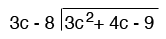First I divide 3c into 3c2 and get c so start the quotient with c and multiply 3c - 8 by c.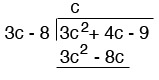Subtract 3c2 - 8c from 3c2 + 4c to get 12c. Bring down -9 to get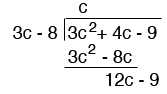At this stage divide 3c into 12c to obtain 4 so the next term in the quotient is 4. Multiply 3c - 8 by 4.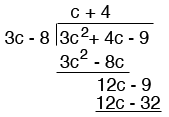Finally subtract -32 from -9 to obtain a remainder of 23.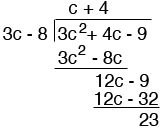Thus 3c2 + 4c - 9 by 3c - 8 is c + 4 with a remainder of 23.

Now try your problem,
HarleyMath Central is supported by the University of Regina and The Pacific Institute for the Mathematical Sciences.